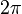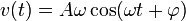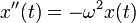# Simple harmonic motion

## Definition

### In terms of the equation for position

Simple harmonic motion is defined as a form of periodic/oscillatory motion along a straight line, with the following form of equation describing the position coordinate as a function of time:$\! x = x(t) = x_0 + A\sin(\omega t + \varphi)$

Here,$x_0$ is the resting position or mean position,$A$ is the amplitude of oscillation (in that it describes the maximum possible magnitude of displacement from the mean position),$\omega$ is a parameter controlling the frequency (in fact,$\omega$ is$2\pi$ times the frequency of completing one full oscillation),$t$ is the time parameter, and$\varphi$ is a phase angle.

As a consequence, we have the following descriptions of the velocity and acceleration functions:$\! v(t) = A\omega \cos(\omega t + \varphi)$

and$\! a(t) = -A\omega^2 \sin(\omega t + \varphi)$

### In terms of the differential equation it solves

Simple harmonic motion is a form of motion of an object/particle along a line subject to the constraint:$\! x''(t) = -\omega^2 x(t)$

where$x(t)$ is the position coordinate of the particle at time$t$ and$x''(t) = a(t)$ is the acceleration (signed) of the particle at time$t$.